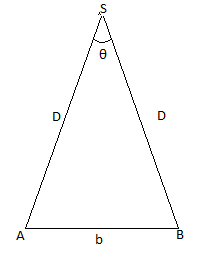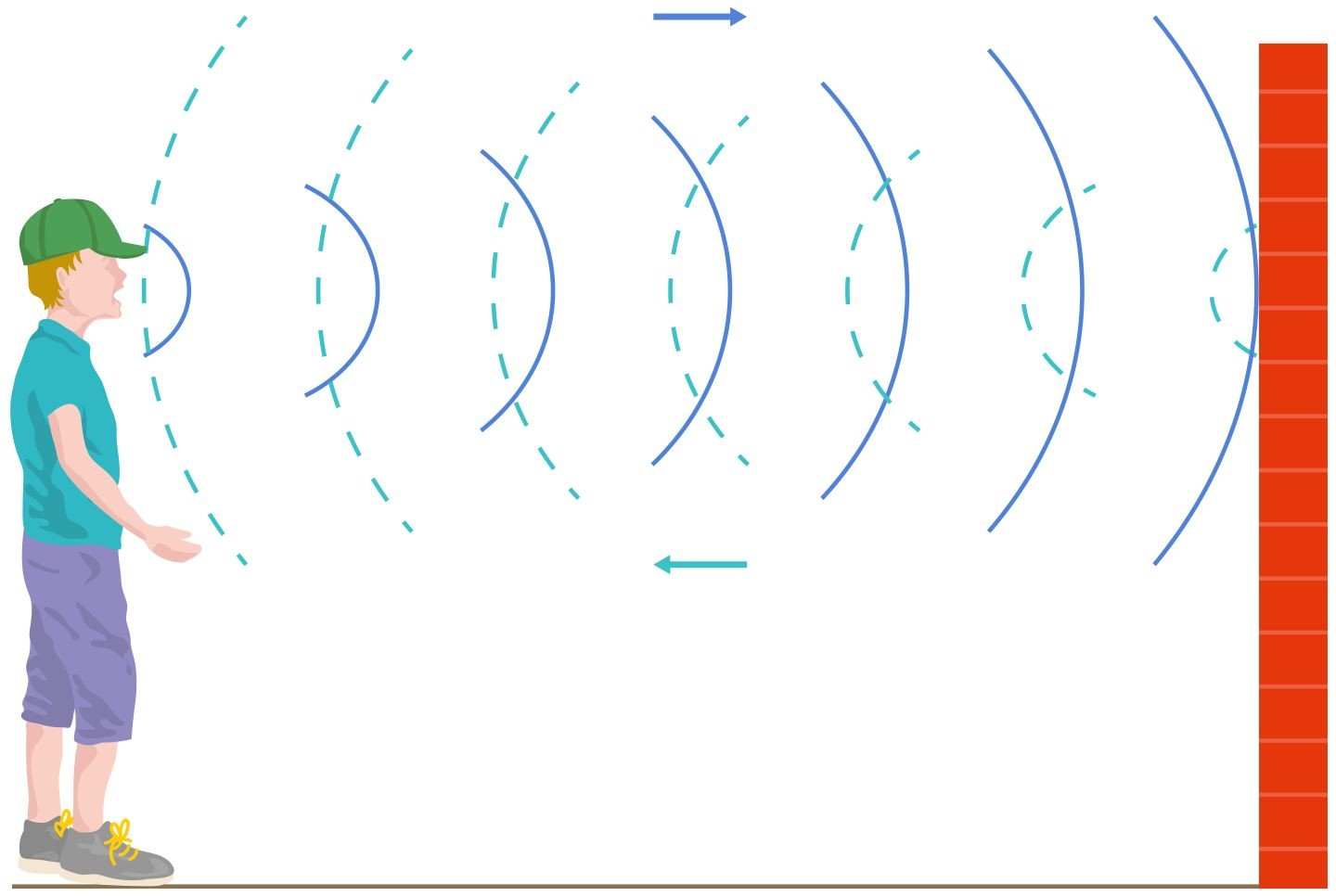×#### Thank you for registering.

One of our academic counsellors will contact you within 1 working day.

Click to Chat

1800-1023-196

+91-120-4616500

CART 0

• 0

MY CART (5)

Use Coupon: CART20 and get 20% off on all online Study Material

ITEM
DETAILS
MRP
DISCOUNT
FINAL PRICE
Total Price: Rs.

There are no items in this cart.
Continue Shopping• Kinematics & Rotational Motion
• OFFERED PRICE: Rs. 636
• View Details

```Measurement of Length

Table of Content

Length

Measurement of Length

Measurement of Large Distance

Parallax Method

Echo Method

Range of Length

Length

Length is defined as the measurement or extent of something from one end to the other end. Length is a scalar quantity and we find it inalmost every fundamental phenomenon in the physical world.

Every experiment in Physics requires the use of length to get desired results. All the external physical measurements rely on length. As an example take your height. How will you measure your height? What is your height? Height is simply the distance between your feet and head. To measure the height you can use either a scale or measure stick.

We need a unit to define the length of something as without unit we won’t be able to compare measure or correlate two objects on the terms of their length. The standard unit of Length is a meter, but being a small unit we refer to big units to make measurements simple. Some examples of the unit are:

Height is measured in “foot” and “inches”, 1 feet contains 12 inches, and 1 inch means 0.0254 meters

Distance is measured in kilometers, 1 kilometer or Km equals to 1000 meter

While performing experiments in Physics lab we prefer small units like millimeter and centimeter, 1 meter = 100 centimeters, and 1 centimeter = 10 millimeters, to avoid writing meter, again and again, we write centimeter as cm, millimeter as mm and decimeter as dm

Metric Conversion Chart

Into Metric
Out of Metric

If you know
Multiply by
To Get
If you know
Multiply by
To Get

Length
Length

Inches
2.54
Centimeters
Millimeters
0.04
Inches

Foot
30
Centimeters
Centimeters
0.4
Inches

Yards
0.91
Meters
Meters
3.3
Feet

Area
Area

Sq. Inches
6.5
Sq. Centimeters
Sq. Centimeters
0.16
Sq. Inches

Sq. Feet
0.09
Sq. Meters
Sq. Kilometers
0.4
Sq. Miles

Sq. Miles
2.6
Sq. Kilometers
Hectares
2.47
Acres

Mass (Weight)
Mass (Weight)

Ounces
28
Grams
Grams
0.035
Ounces

Pounds
0.45
Kilograms
Kilograms
2.2
Pounds

Short ton
0.9
Metric Ton
Metric Tons
1.1
Short Tons

Volume
Volume

Teaspoons
5
Milliliters
Milliliters
0.03
Fluid Ounces

Tablespoons
15
Milliliters
Liters
2.1
Pints

Fluid Ounces
30
Milliliters
Liters
1.06
Quarts

Cups
0.24
Liters
Liters
0.26
Gallons

Pints
0.47
Liters
Cubic Meters
35
Cubic Feet

Quarts
0.96
Liters
Cubic Meters
1.3
Cubic Yards

Cubic feet
0.03
Cubic Meters

Cubic Yards
0.76
Cubic Meters

Temperature
Temperature

Fahrenheit
Subtract 32, then multiply by 5/9 ths to get
Celsius
Celsius
Multiply by 9/5ths, then add 32 to get
Fahrenheit

We measure length in certain units, according to our preference which makes our measurement more precise and accurate. Units are always chosen to make measurements simple and to make it error free.

Measurement of Length

We know some direct methods of measuring length. There are several kinds of instruments we use to measure the length of objects. For example, when we were kids we used centimeter scale to draw lines and measure the length of small objects. When the nature of measuring objects change, we switch to new instruments. Some examples includeWe use Vernier caliper to measure lengths of order 10-4m

We use meter scale to measure lengths from 10-3m to 102m

We use a screw gauge to measure lengths of order less than 10-5m

There are some objects which can’t be measured with the help of scales and instruments, like the height of the mountain, the radius of earth or other large bodies. To measure them, we use some special methods.

Measurement of Large Distance

To measure large distances like the distance of a planet from earth or sun, we can’t use meter scale. Instead, we use some special methods, which can make complex measurements simple. There are two such methods, which we use to measure infinitely large distances.

Parallax Method

Echo Method

Parallax Method

Parallax Method is used to measure large distances and works on the principle of parallax basis. Parallax is defined as the apparent displacement of an object when observer’s point of view changes.Example:

Hold a pencil in your hand. Now close your left eye and see the pencil, now close your right eye and see the pencil. You’ll find that position of theimage of the pencil is different in each case. This is called Parallax and your eyes are used as a basis.

To measure a distance D of point A from a planet S by parallax method, we will observe it from two different views A and B. The distance between A and B is b. The angle ASB or θ is called parallax angle or parallactic angle.

As the distance between planet and observation points is very large, we can assume

b/D << 1

which means, angle θ to be very small. We proceed further by taking the triangle ASB in form of arc, where the length of the arc is b and radius of the arc are D.

Arc length = Radius × Angle θ

b = D × θ

Now if we know the distance between two points and parallax angle, we can easily find the distance D, with the help of above relation.

Example

A planet is observed from two points A and B on earth, the angle θ is 3.32 × 10-2 radian. The diameter of the earth is about 1.276 × 107m. Find out the distance between Earth and Moon.

We have θ = 3.32 × 10-2 radian, taking two points on a diameter of the earth, we have b = 1.276 × 107m. The unknown quantity is D,

Now since D = b/ θ

D = 1.276 × 107m/ 3.32 × 10-2

D = 3.84 × 108 m

Echo Method

Echo Method is used to find the distance of a hill or building from a certain point on the surface of the earth. In this method, we shoot a gun or radio wave and we measure the time interval in which gun or radio wave is fired and instant echo is heard.If t is the time taken by the sound to travel from observer to the hill and back to the hill, and S be the distance between observer and hill then

We know that

Speed = Distance/ Time

v = 2S/t

(Since the total distance covered by sound will be double)

If we know the speed of sound, we can easily find the distance between the observation point and hill. This is called Echo Method.

Range of Length

The sizes of objects and heavenly bodies vary over a wide range. The length of organisms affects at both macroscopic and microscopic levels. For example, the size of the universe is of the order 1026m whereas the size of a tiny nucleus of an atom is just 10-14m. We use certain units to define length at both levels. They are:

1 fermi or 1f equals to 10-15m

1 Angstrom or 1Å equals to 10-10m

1 Astronomical unit or AU is 1.496 × 1011m (it is also the average distance between the sun and the earth)

1 light year or 1ly equals to 9.46 × 1015m (it is the distance which light travels with velocity of 3 × 108 m/s in 1 year)

The table below depicts range and order of different lengths.

Distance or Size of object
Length (m)

Size of proton

10-15

Size of atomic nucleus

10-14

Size of hydrogen atom

10-10

Length of typical virus

10-8

Wavelength of light

10-7

Size of red blood corpuscle

10-5

Thickness of paper

10-4

Height of Mount Everest above sea level

104

107

Distance of Moon from Earth

108

Distance from Sun from Earth

1011

Distance of Pluto from Sun

1013

Size of our galaxy

1021

Distance to Andromeda galaxy

1022

Distance to boundary of observable universe

1026

Watch this Video for more reference

Measurement of Length
```### Course Features

• 731 Video Lectures
• Revision Notes
• Previous Year Papers
• Mind Map
• Study Planner
• NCERT Solutions
• Discussion Forum
• Test paper with Video Solution